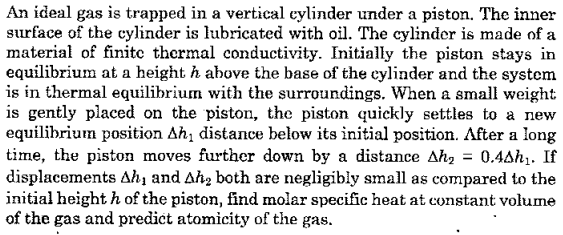# Can anyone please tell me from which Russian Olympiad are these questions from?

Идеальный газ находится в вертикальном цилиндре под поршнем. Внутренняя поверхность цилиндра смазывается маслом. Цилиндр изготовлен из материала с конечной теплопроводностью. Первоначально поршень находится в равновесии на высоте h над основанием цилиндра, и система находится в тепловом равновесии с окружающей средой. Когда на поршень осторожно помещают небольшой груз, поршень быстро опускается в новое положение равновесия на расстояние Δh1 ниже своего исходного положения. По истечении длительного времени поршень перемещается дальше вниз на расстояние Δh2 = 0,4Δh1• Если оба перемещения Δh1 и Δh2 пренебрежимо малы по сравнению с начальной высотой h поршня, найти молярную теплоемкость при постоянном объеме газа и предсказать атомарность газа.

I am also attaching an image of the question. The question is from an olympiad booklet in India.1 симпатия

sorry, but i haven’t seen such question before

1 симпатия

Ok… so that means this question is original and maybe not taken from any olympiad. It is a very difficult problem.

Хорошо… так что это означает, что этот вопрос является оригинальным и, возможно, не взят из какой-либо олимпиады. Это очень трудная проблема.

well, at least you may ask here for help to solve this problem2 симпатии

Thank you! And yeah, please help in this problem… I was unable to solve this even after attempting it several times.

Благодарю вас! И да, помогите пожалуйста с этой проблемой…

1 симпатия

So here you can write the ideal gas law

p_0 Sh = \nu RT_0,

where p_0 is an atmospheric pressure and T_0 is a surface temperature (because at the start the system was both in mechanical and thermal equilibrium with the surroundings).

Then we place a small weight, giving an additional pressure mg/S to system (we don’t know the mass but further we can calculate it). Sentence “The piston quickly settles to a new equlubrium position \Delta h_1 distance below its initial position” implies that after reaching \Delta h_1, the system stays in mechanical, though not thermal equlibrium with the surface (well, oscillations of the piston also take place in this process here, but let’s think that the oscillation time is much smaller than specific time for system to cool down). So we use 1st thermodynamic law

Q = A+\Delta U,

but in this process Q=0. Also, adiabatic equation pV^\gamma=\text{const} does not work by the reason that this process is not quasistatic. How to calculate work A? Well, we can do it in terms of external pressure:

-A = (p_0+\frac{mg}{S})\cdot S\Delta h_1.

Here’s the “minus” sign before A because this is the work of external pressure. So,

\Delta U = \nu C_V(T_1-T_0) = (p_0S+mg)\Delta h_1,

and it is understood that T_1>T_0, so next the piston cools down until system reaches temperature T_0. But before cooling down, we may write another ideal gas law

(p_0 + \frac{mg}{S})S(h-\Delta h_1) = \nu RT_1,

and after a long time,

(p_0S+mg)(h-\Delta h_1 - \Delta h_2) = \nu RT_0

Then we can find C_V. Using equation for \Delta U (and expressing T_0 and T_1 from ideal gas equations),

C_V = R\cdot\frac{(p_0S+mg)\Delta h_1}{\nu RT_1-\nu RT_0} = R\cdot \frac{(p_0S+mg)\Delta h_1}{(p_0S+mg)(h-\Delta h_1) - (p_0 S + mg)(h - \Delta h_1 - \Delta h_2)}=R\cdot\frac{\Delta h_1}{\Delta h_2}=\frac{5}{2}R

Thus, gas is diatomic.

6 симпатий

also, we can’t always tell where are these problems actually from, because we’re not from Russia; there are mostly students from Kazakhstan in this forum. but still you can ask your questions and we will answer them

2 симпатии

Thanks a lot for your help! I actually did not know that most of the students aren’t from Russia…but I’ll remember it in future.

5 симпатий